# VBA Convert Text String to Number

You may be required to convert numbers stored as text to actual numbers in your VBA code. In this tutorial, we are going to go over the functions that you need to use to convert a string to an integer, long, double, decimal or currency data type (Click here to learn about converting numbers to strings)

## Convert String to Integer

You can use the CInt or CLng function to convert a string to an integer. If the fraction is less than .5 the function will round down, if the fraction is greater than or equal to .5 the function will round up. The following code will convert a string to an integer:

``MsgBox CInt("7.55")``

The result is: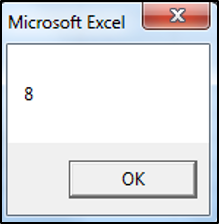The following code uses the CLng function to convert a string to an integer:

``MsgBox CLng("13.5")``

The result is: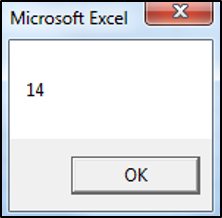Note: You can use the CInt or CLng function to convert a string to an integer or long (respectively) data types. The Long Data type is the same as an integer data type except larger numbers are allowed. In the past, the distinction was required because of memory constraints. In modern programming, there’s no reason not to use the long data type since memory is no longer an issue. So it’s always better to use a long data type instead of an integer.

You can use the Immediate Window to see how the value would be processed if not converted to an integer:

``Debug.Print "13.5" + "13.5"``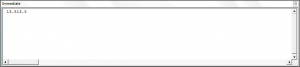Usually, the text will be stored as a variable and this variable will need to be converted to a number data type as shown in the code below:

``````Sub Using_Variables()

Dim valueOne As String
valueOne = 5
MsgBox CLng(valueOne) + CLng(valueOne)

End Sub``````

## Convert String to Decimal

You can use the CDbl or CDec function to convert a string to a decimal. The following code would convert a string to a double data type:

``MsgBox CDbl("9.1819")``

The result is: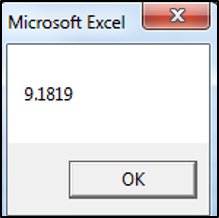The following code would convert a string to a decimal data type:

``MsgBox CDec("13.57") + CDec("13.4")``

The result is: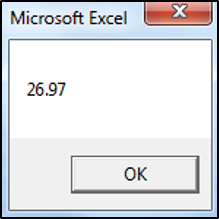You can use the Immediate Window to see how the value would be processed if not converted to a double or decimal data type:

``Debug.Print "13.57" + "13.4"``

The result is: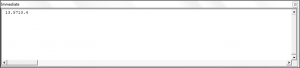Note: The decimal data type can store larger numbers than the double data type, so it’s always advisable to use the decimal data type when you are uncertain.

## Convert String to Currency

You can use the CCur function to convert a string to a currency. The following code would convert a string to a currency data type:

``Range("A1").Value = CCur("18.5")``

The result is: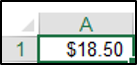Stop searching for VBA code online. Learn more about AutoMacro - A VBA Code Builder that allows beginners to code procedures from scratch with minimal coding knowledge and with many time-saving features for all users!